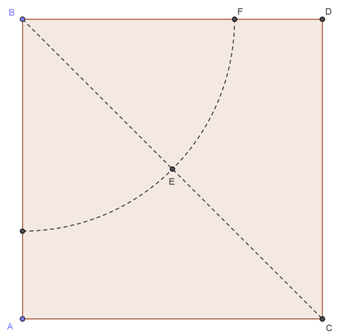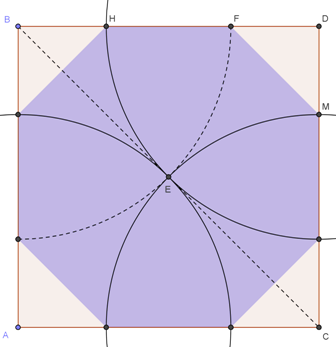Medieval stonemasons used this method to construct octagons in a given square window.

Open your compasses to a radius of half the diagonal of the square and construct an arc with centre one vertex of the square - mark the two points where the arc crosses the sides.Do that for all 4 vertices of the square giving 8 points which are the vertices of an octagon.Is the octagon regular?

Can you prove it?